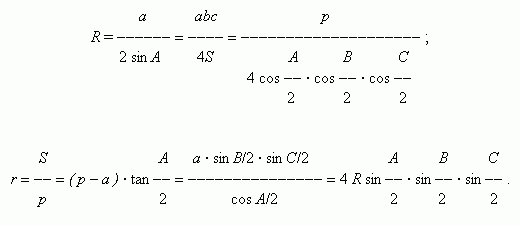# Basic relations between elements of triangle

Law of cosines. Law of sines. Law of tangents.
Area formulas. Heron's  formula.
Radii of circumscribed and inscribed circles.

Designations: a , b , c – sides; A , B , C – angles of triangle; p = ( a + b + c ) / 2  - a half-perimeter; h – a height; S – an area; R , r –  radii of circumscribed and inscribed circles correspondingly.

Law of cosines: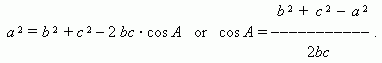Law of sines: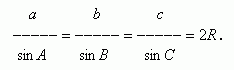Law of tangents: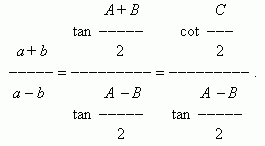Area formulas: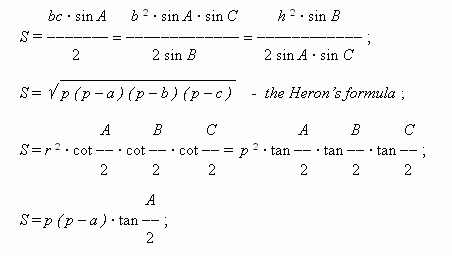Radii of circumscribed and inscribed circles: